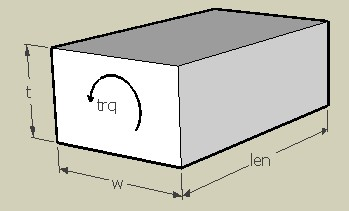TORBAR,len,w,t,trq,ymod,pois,sel
Design of a torsion bar beam suspension

len         length of the beam in µm
w           length of longer side of the beam in µm
t            length of the shorter side of the beam in µm
trq         applied torque in  µNµm
ymod    Young's modulus of the material of the beam in GPa
pois       Poisson's ratio
sel         number denoting the selected result.
Use 1 for torsional spring constant and 3 for maximum shear stressNotes

The rectangle shaped torsion bar beam suspension is often used in MEMS where a twisting or turning movement of the proof mass is required like that in a micro mirror or accelerometer. The beam twists under an applied torque till the restoring torque of the beam balances the applied torque.

This design interface can be used to determine the torsion constant and maximum shear stress of a rectangular shaped torsion bar. Torsion constant is defined as the restoring torque per unit angular displacement. The maximum shear stress happens at the middle of the longer side of the crosssection.

The stiffness of a single torsion bar is calculated.  If the spring constant of one beam is k1 and the second spring is k2, and if they are connected in parallel, the effective spring constant

Kparallel = k1 + k2.

The plot shows the dependency of torsional spring constant on the cross-sectional dimension of the beam. The X axis of the plot is the ratio of the length of the longer side of the cross section of the beam to its shorter side (w/t) in a logarithmic scale. It shows that the torsional stiffness increases rapidly with this ratio. This is for a single torsion bar. The longer side of the beam can be made its thickness, to reduce the out of plane movement.

Assumptions

-Spring constant of a single beam is calculated
-Shearing effects are considered, axial deformations are assumed to be negligible.
-The default material is Silicon with a Young's modulus of 180GPa and Poisson's ratio of 0.3.
-The beam has uniform rectangular cross section.
-The weight of the beam is uniformly distributed.
-In single beam analysis, the point where the mass is attached is assumed to be free to move.